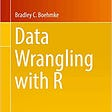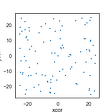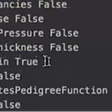# What is support vector regression (SVR) ?

## An introduction to machine learning algorithms

A support vector regression is a popular machine learning model today in this article, I would be giving you a detailed explanation and how this model works.

support vector regression comes in the field of supervised learning.

# Support vector machine and regression

Support vector model can be used for both problems regression as well as classification and it’s divided into 2 parts support vector machine (SVM)is used for classification problems and support vector regression (SVR) is mostly used for regression problems but in this article, I would be telling you about support vector regression (SVR) to know more about support vector machine (SVM) go to this link

so first of all

## What is a hyperplane?

the hyperplane is the boundary between two classes that separates them from each other and the hyperplane can be dependent on the dataset if our data is 2 dimensional the hyperplane will be a line and if our data is 3 dimensional then the hyperplane will be a 2d plane which separates the data points

## How does it work

SVR finds a hyperplane in an n-dimension or a line to

classify between the dataset so when we add a new point in the dataset the SVM can tell what class the point belongs to

# linear SVR

linear SVR when our data is linear then linear SVR and we can separate them from a straight line

# non-linear SVR

non-linear SVR when our data is non-linear then we use non-linear SVR and it is not separable by a straight line but instead can be separated by a curve

# Example

## Linear SVR

in linear SVR when our data looks like this we can plot a line to make a relationship so we will plot a line between our dataset so we can get a relation between the dataset then there are many lines we can draw that can pass between all of the points in our data but to find the best line

we need to introduce a hyperplane which consists of mostly all the lines which separates the data and support vectors helps them to build the hyperplane

## Non-linear SVR

non-linear SVR in the linear SVR we used a line to find the relationship between our data but in non-linear SVR when our data looks something like this and cannot be separated by a line we need to introduce a curve to find the relationship between the data

# conclusion

so I hope today you guys have a good understanding of support vector regression in the near future I would be making more articles in which I will be explaining more models.

--

--

--

## More from Aviral Bhardwaj

writer

Love podcasts or audiobooks? Learn on the go with our new app.

## Finding Your Next Beloved Artists## Why do organisations need a data strategy?## The Story of Data — Issue 1## PyNetlogo Tutorial## Found This Week #51## Business Intelligence moving towards collaboration## What Exactly is Data Stewardship and Why Do You Need It?writer

## Diabetes Prediction Using Machine Learning Algorithms## Lets Understand Bias and Variance Trade off…….## Predicting Class of Skin Lesions## Introduction to Machine Learning